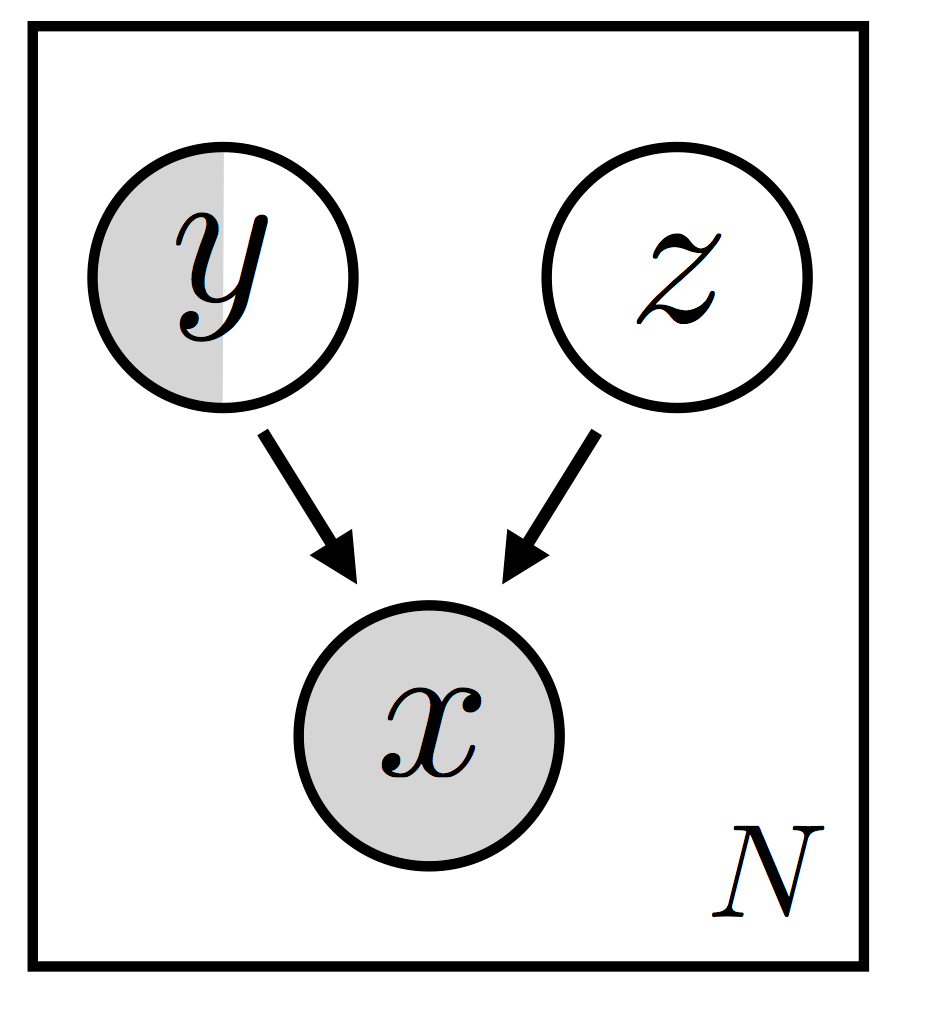# Causal Generative Modelling — A Brief Tutorial with Game Character Images

Original article was published by Harish Ramani on Deep Learning on MediumImplementation

Technology stack: Pyro (probabilistic programming language from uber) , pytorch, bnlearn and gRain (R packages to deal with bayesian network structure learning)

All probabilistic programs are built up by composing primitive stochastic functions and deterministic computation. In our case, the data generating process is encoded into the DAG and is implemented to a stochastic function, conventionally named, model. In this stochastic function, we define each of the nodes in the DAG to be sampled from a specific distribution. In our case, all the nodes except the image are discrete variables and hence sampled from a categorical distribution. Since we have training labels we can use them in conjunction while sampling from the nodes. If there are any learn-able parameters, as in our case the encoder and decoder neural networks, we need another stochastic function named guide to help learn these parameters. Inference algorithms in pyro, such as stochastic variational inference, use the guide functions as approximate posterior distributions. Guide functions must satisfy two criteria to be valid approximations of the model. One, all the unobserved sample statements that appear in the model must appear in the guide. Second, the guide has the same signature as that of the model, i.e. it takes the same arguments.

`actor = pyro.sample("actor",     dist.OneHotCategorical(self.cpts["character"]), obs=actorObs)`

Here, we sample the actor from a categorical distribution, one hot encoded with a certain prior probability as mentioned in the CPT section and condition with the observed label, using the obs argument.

In the guide function, we sample unobserved nodes of the DAG. In our example, we don’t observe the strength, attack, and defense attributes of the actor and the reactor in the image. Hence, we use the guide function and learn their posterior distributions. We compute the conditional probability of the unobserved nodes, indexed by the values of their parents and children nodes. For actor_strength, the parents of the node are actor and actor type and the children are action. These 3 entities are observed in our training data.

One of the guide statement is mentioned below

`actor_strength = pyro.sample("actor_strength",    dist.Categorical(    self.inverse_cpts["action_strength"][action, actor_type,actor]))`

Note: Please refer the DAG if you’re confused. The variables in guide function are inferred by taking the parents and the children nodes of the variable.

Variational Auto Encoder Architecture

Variational Autoencoders are directed probabilistic graphical models whose posteriors are approximated by a neural network with an autoencoder like architecture. The Autoencoder architecture comprises of an encoder unit, which reduces the large input space to a latent domain, usually of lower dimension than of input space, and a decoder unit which reconstructs the input space from the latent representation.

The brief outline of the causal variational autoencoder network is given below.

Causal VAE higher outline

The Encoder and Decoder have Convolution Units as we deal with images and we use Stochastic Variational Inference to train this model.

Inference Mode

In inference mode, we use the trained decoder network in conjugation with the latent node to generate an image for various probabilistic queries. In inference mode, instead of doing inference using MCMC or HMC, we pre-compute the posterior distributions using analytic methods using the gRain package in R. This is not possible in all cases.